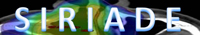Linear quadratic model : D2 = D1(α/β + d1) / (α/β + d2) where D2 = equivalent total dose, D1 = initial total dose, d1 = initial dose / fraction and d2 = wanted dose / fraction   Several factors should be considered before using the Linear Quadratic Model : - Dose / fraction : must be between 1 and 6 Gy. The linear quadratic model should not be used for hypofractionated regimen. - Time between fractions : Two treatment sessions should not be performed with less than 6 to 8 hours between them. The Linear Quadratic Model should not be used for accelerated treatments.© - SFJRO - 2010